Courses

# NCERT Solutions(Part- 2)- Linear Equations in One Variable Class 8 Notes | EduRev

## Mathematics (Maths) Class 8

Created by: Vp Classes

## Class 8 : NCERT Solutions(Part- 2)- Linear Equations in One Variable Class 8 Notes | EduRev

The document NCERT Solutions(Part- 2)- Linear Equations in One Variable Class 8 Notes | EduRev is a part of the Class 8 Course Mathematics (Maths) Class 8.
All you need of Class 8 at this link: Class 8

EXERCISE 2.2

Ques 1. If you subtract 1/2 from a number and multiply the results by 1/2, you get 1/8. What is the number?

Ans: Let the required number be x.

∴ According to the condition, we have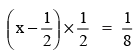Multiplying both sides by 2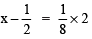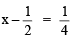Adding 1/2 both sides, we have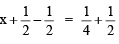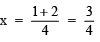∴ The required number = 3/4

Ques 2. The perimeter of a rectangular swimming pool is 154 m. Its length is 2 m more than twice its breadth. What are the length and the breadth of the pool?

Ans: Perimeter of the rectangular pool = 154 m
∴ Length = 2(breadth) + 2 metres = (2x + 2) metres.
Since, perimeter of a rectangle = 2(length + breadth)

∴ 2[(2x + 2) + x] = 154
Dividing both sides by 2

⇒ (2x + 2) + x = 154/2 = 77
⇒ 3x + 2 = 77

Transposing 2 to RHS, we have
3x = 77 – 2 = 75
Dividing both sides by 3, we have

x = 75/3 =  25
Length = 2(25) + 2 = 52 m.

Ques 3. The base of an isosceles triangle is 4/3 cm. The perimeter of the triangle is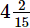cm. What is the length of either of the remaining equal sides?

Ans: Base of the isosceles triangle = 4/3 cm.
Let the length of either equal sides = x cm.

∴ Perimeter of the triangle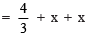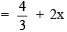But the perimeter of the triangle =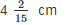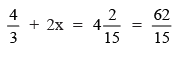Transposing 4/3 to RHS, we have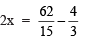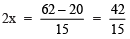Dividing both sides by 2, we have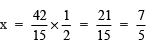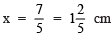Thus, the required length =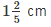Ques 4. Sum of two numbers is 95. If one exceeds the other by 15, find the numbers.

Ans: Let the smaller number = x.

The other number = x + 15.
According to the condition, we have

x + [x + 15] = 95
2x + 15 = 95
or  2x = 95 – 15 = 80

Dividing both sides by 2, we have

x = 80/2 = 40
Therefore, The smaller number = 40
and the other number = 40+15 = 65

Ques 5. Two numbers are in the ratio 5 : 3. If they differ by 18, what are the numbers?

Ans: Let the two numbers be 5x and 3x.

According to the condition, we have

5x – 3x = 18
⇒ 2x = 18

Dividing both sides by 2, we have

2x/2 = 18/2
⇒ x = 9
∴  5x = 5 × 9 = 45 and 3x = 3 × 9 = 27
Thus, the required numbers are 45 and 27.

Ques 6. Three consecutive integers add up to 51. What are these integers?

Ans: Let consecutive numbers be x, x + 1 and x + 2.

According to the condition, we have

x + [x + 1] + [x + 2] = 51
or  x + x + 1 + x + 2 = 51
or  3x + 3 = 51

Transposing 3 to RHS, we have
3x = 51 – 3 = 48

Dividing both sides by 3, we have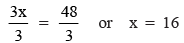Now x + 1 = 16 + 1 = 17
and x + 2 = 16 + 2 = 18

Thus, the required three consecutive numbers are: 16, 17 and 18.

Ques 7. The sum of three consecutive multiples of 8 is 888. Find the multiples.

Ans: Let the three multiples of 8 are: x, x + 8 and x + 8 + 8 = x + 16.

According to the condition, we have

[x] + [x + 8] + [x + 16] = 888

or  x + x + 8 + x + 16 = 888
or  3x + 24 = 888

Transposing 24 to RHS, we have

3x = 888 – 24 = 864

Dividing both sides by 3, we have

x = 864/3 = 288

∴ x + 8 = 288 + 8 = 296 and x + 16 = 288 + 16 = 304

Thus, the required multiples of 8 are: 288, 296 and 304.

Ques 8. Three consecutive integers are such that when they are taken in increasing order and multiplied by 2, 3 and 4 respectively, they add up to 74. Find these numbers.

Ans: Let the integers be x, (x + 1) and (x + 2).
∴ According to the condition, we have

2 × (x) + 3 × (x + 1) + 4 × (x + 2) = 74
⇒ 2x + 3x + 3 + 4x + 8 = 74
⇒ 9x + 11 = 74
⇒ 9x = 74 – 11 = 63

Dividing both sides by 9, we have

x = 63/9 = 7
∴ The required integers are: 7, (7 + 1) and (7 + 2) or 7, 8, and 9.

Ques 9. The ages of Rahul and Haroon are in the ratio 5 : 7. Four years later the sum of their ages will be 56 years. What are their present ages?

Ans: Since, ages of Rahul and Haroon are in the ratio of 5 : 7.
Let their present ages are: 5x and 7x.
∴ 4 years later:
Age of Rahul = (5x + 4) years
Age of Haroon = (7x + 4) years

According to the condition, we have
(5x + 4) + (7x + 4) = 56
or   5x + 4 + 7x + 4 = 56
or  12x + 8 = 56

Transposing 8 to RHS, we have

12x = 56 – 8 = 48

Dividing both sides by 12, we have
x = 48/12 = 4
∴ Present age of Rahul = 5x = 5 × 4 = 20 years

Present age of Haroon = 7x = 7 × 4 = 28 years

Ques 10. The number of boys and girls in a class are in the ratio 7 : 5. The number of boys is 8 more than the number of girls. What is the total class strength?
Ans: Since, [number of boys] : [number of girls] = 7 : 5
Let the number of boys = 7x
and the number of girls = 5x
According to the condition, we have
7x = 5x + 8
Transposing 5x to LHS, we have

7x – 5x = 8

or  2x = 8

Dividing both sides by 2,

x = 8/2 = 4

∴ Number of boys = 7x = 7 × 4 = 28
Number of girls = 5x = 5 × 4 = 20

∴ Total class strength = 28 + 20 = 48 students.

Ques 11. Baichung’s father is 26 years younger than Baichung’s grandfather and 29 years older than Baichung. The sum of the ages of all the three is 135 years. What is the age of each one of them?
Ans: Let the age of Baichung = x years
∴  His father’s age = (x + 29) years
⇒ His grandfather ’s age = (x + 29 + 26) = (x + 55) years

According to the condition, we have

[x] + [x + 29] + [x + 55] = 135 years
or  x + x + 29 + x + 55 = 135
or  3x + 84 = 135

Transposing 84 to RHS, we have

3x = 135 – 84

or 3x = 51

Dividing both sides by 3, we have
x = 51/3 = 17

Now, Baichung’s age = 17 years

His father’s age = 17 + 29 = 46 years

His grandfather ’s age = 46 + 26 = 72 years.

Ques 12. Fifteen years from now Ravi’s age will be four times his present age. What is Ravi’s present age?

Ans: Let Ravi’s present age = x years
4 times the present age = 4 × x = 4x years
15 years from now, Ravi’s age = (x + 15) years

According to condition, we have

x + 15 = 4x

Transposing 15 and 4x, we have

x – 4x = –15
or  –3x = –15

Dividing both sides by (–3), we have

x = (–15)/(–3) = 5

∴ Ravi’s present age = 5 years.

Ques 13. A rational number is such that when you multiply it by 5/2 and add 2/3 to the product,you get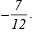. What is the number?

Ans: Let the required rational number be x.

∴ According to the condition, we have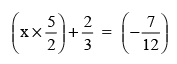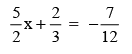Transposing 2/3 to RHS, we have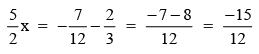Dividing both sides by 5/2, we have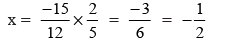The required integer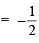Ques 14. Lakshmi is a cashier in a bank. She has currency notes of denominations Rs 100, Rs 50 and Rs 10, respectively. The ratio of the number of these notes is 2 : 3 : 5. The total cash with Lakshmi is Rs 4,00,000. How many notes of each denomination does she have?

Ans: Let the number of:
Rs 100-notes = 2x
Rs 50-notes = 3x
Rs 10-notes = 5x
∴ Value of Rs 100-notes = 2x × Rs 100 = Rs 200x
Value of Rs 50-notes = 3x × Rs 50 = Rs 150x
Value of Rs 10 we have 10-notes = 5x × Rs 10 = Rs 50x

According to the condition, we have
Rs 200x + Rs 150x + Rs 50x = Rs 4,00,000
or 200x + 150x + 50x = 400000
or 400x = 400000

Dividing both sides by 400, we have

x = 400000/400 = 1000
Therefore, 2x = 2000 = Rs 100-notes
3x = 3000 = Rs-50 notes
5x = 50000 = Rs-10 notes

Ques 15. I have a total of Rs 300 in coins of denomination Re 1, Rs 2 and Rs 5. The number of Rs 2 coins is 3 times the number of Rs 5 coins. The total number of coins is 160. How many coins of each denomination are with me?
Ans:
Let the number of Rs 5 coins = x
∴  The number of Rs 2 coins = 3x
∵ Total number of coins = 160
∴  Number of Re 1 coins = 160 – 3x – x
= (160 – 4x)

Now, value of:
Rs 5 coins = Rs 5 × x = Rs 5x
Rs 2 coins = Rs 2 × 3x = Rs 6x
Rs 1 coins = Rs 1 × (160 – 4x) = Rs (160 – 4x)

According to the condition, we have

5x + 6x + (160 – 4x) = 300
or 5x + 6x + 160 – 4x = 300
or 7x + 160 = 300

Transposing 160 to RHS, we have
7x = 300 – 160 = 140

Dividing both sides by 7, we have

x = 140/7 = 20
∴ Number of Rs 5-coins = 20
Rs 2-coins = 3 × 20 = 60
Rs 1-coins = 160 – (20 × 4) = 80

Ques 16. The organizers of an essay competition decide that a winner in the competition gets a prize of Rs 100 and a participant who does not win gets a prize of Rs 25. The total prize money distributed is Rs 3,000. Find the number of winners, if the total number of participants is 63.

Ans: Let the number of winners = x
∴ Number of participants who are not winners = (63 – x)
∴ Prize money given to: Winners = x × Rs 100 = Rs 100x
Non-winner participants
= Rs 25 × (63 – x)
= Rs 25 × 63 – Rs 25x
= Rs 1575 – Rs 25x

According to the condition, we have

100x + 1575 – 25x = 3000
Transposing 1575 to RHS
⇒ 75x = 3000 – 1575

75x = 1425
Dividing both sides by 75

x = 1425/75 = 19

Thus, the number of winners = 19

Offer running on EduRev: Apply code STAYHOME200 to get INR 200 off on our premium plan EduRev Infinity!

## Mathematics (Maths) Class 8

212 videos|147 docs|48 tests

,

,

,

,

,

,

,

,

,

,

,

,

,

,

,

,

,

,

,

,

,

;